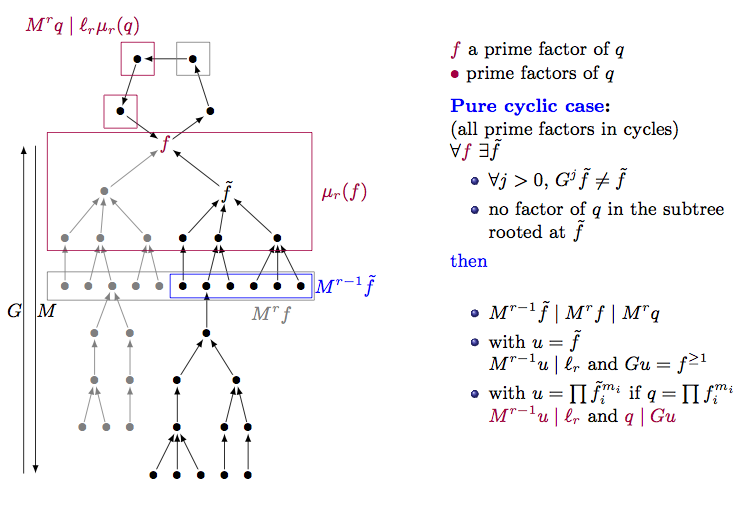PhD's web siteMain Publications Books SpecFun TeamMy main topic of research is about divide-and-conquer recurrences. More formally, I am interested in sequences that are rational with respect to a numeration system, either from an asymptotic standpoint or in relation with Mahlerian functional equations.The image illustrates the search for rational solutions of linear Mahler equations, more precisely the search for a common denominator $q$ of such solutions. In case of recurrence equations, the useful polynomials are on lines. Here lines are replaced by trees. Moreover there can be cycles. The vertical arrows indicate the action of the Mahler operator $M$ and the Gräffe operator $G$ on prime polynomials. Linear divide-and-conquer recurrences translate into linear Mahler equations for the generating functions of the sequences. For example the recurrence for the Karatsuba algorithm complexity $u_n = u_{\lfloor n/2 \rfloor} + u_{\lceil n/2 \rceil} + n - 1$ translates into $u(x) = \frac{(1+x)(2+x)}{x} u(x^2) - x + \frac{4 x^2}{(1-x)^2}.$ The search for solutions of these functional equations in some classes of functions is motivated by two reasons. First there is a general trend to solve functional equations, that has begun with the work of Liouville (1833) about differential equations and was continued by Abramov (1989) for recurrences. Particularly the search for rational solutions is a building block for other algorithms (like summation algorithms). Next Mahler equations are related to transcendence issues. This standpoint comes from Mahler's work (1929, 1930) about this topic. Recently several works (Adamcwezski and Faverjon, 2015; Dreyfus, Hardouin, and Roques, 2015, among others) provide criteria to recognize if an object is transcendental and these criteria are based on the existence of solutions of a given type for some Mahler equations. This leads to the writing of an article Computing solutions of linear Mahler equations, 2016, you can find in the page devoted to my publications.

 postal address: Philippe Dumas INRIA Saclay Bâtiment Alan Turing 1 rue Honoré d'Estienne d'Orves Campus de l'École Polytechnique 91120 Palaiseau FRANCE email address: Firstname.Lastname@inria.fr